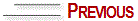# CONSTRUCTION OF CUBES IN A SPACE UNDER A SINGLE VIEW POINT – MEASURED IN MILLIMETERS IN PARALLEL PERSPECTIVE

See figure nº 12 (next page).

Construct the visual space with the distance, horizon and support lines and place the respective view, vanishing and depth points. Draw squares in the height and dimensions desired. See figure nº 1. Find the depth of the box (figure nº 2). Construct the planes of the box with 2 horizontal lines (figure nº 3). See the constructed cube in figure nº 4. In figure nº 5 we can see the construction of a sequence of squares in depth. It is important to note that the dimensions of the horizontal planes found with the diagonal line that goes from the vertex of the square to the depth point are identical to the vertical square of the first plane.© Yvonne Tessuto Tavares - All rights reserved